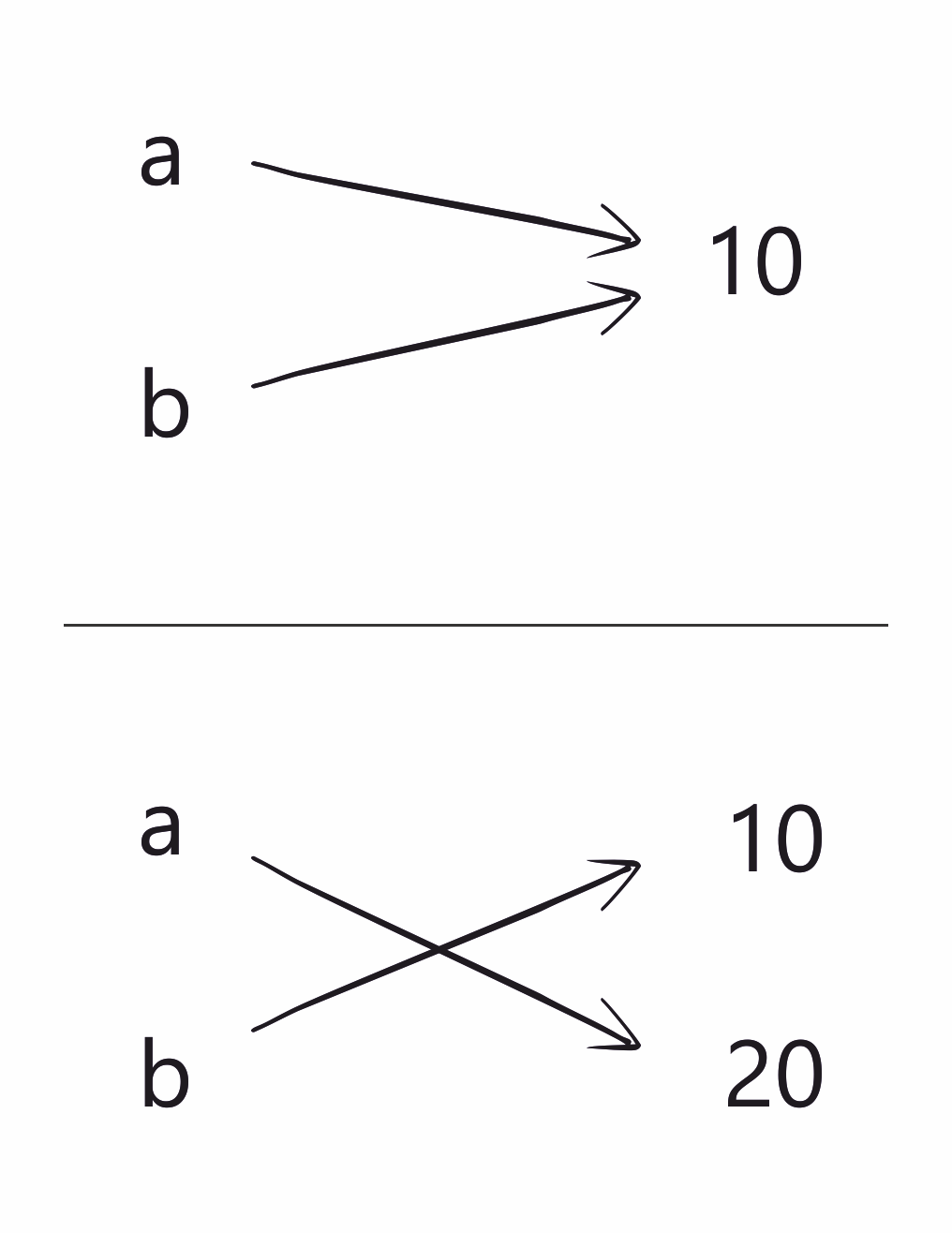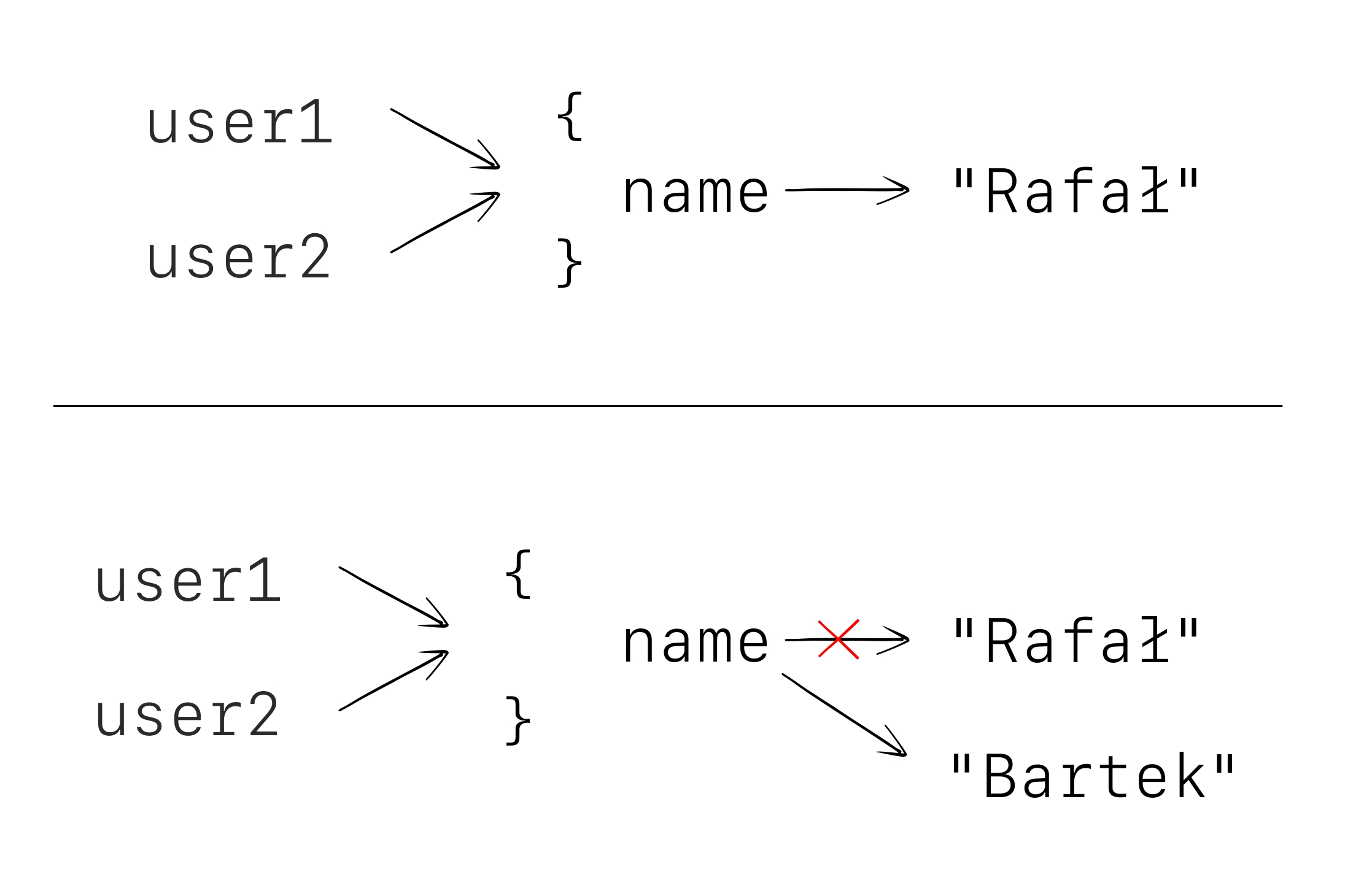# Variables point to objects

There is one thing, that is typically thaught on basic courses for progreamming languages, but many people get it wrong. Then I often see even senior developers who make mistakes on code snippets that are simple for some of developers who are just learning. Take a look at the following snippet.

var a = 10 var b = a a = 20 println(b)

What will be printed? The answer is 10. To understand it, it is best to think of variables like a pointers pointing to same object. It is always an object, never another variable. First a points to 10, then b points to 10, then a changes and points to 20. This does not change that b points to.This picture gets more complicated when we introduce mutable objects. Take a look at the following snippet.

val user1 = object { var name: String = "Rafał" } val user2 = user1 user1.name = "Bartek" println(user2.name)

What will be printed? The answer is "Bartek". Here both user1 and user2 point to the same object, and then this object changes internally.The situation would be different, if we would change what is user1 pointing to, instead of changing the value of the name.

var user1 = object { var name: String = "Rafał" } val user2 = user1 user1 = object { var name: String = "Rafał" } println(user2.name)

What is the answer? It is "Rafał" now.

This is especially confusing if we compare mutable lists with read-only lists referenced with var. Especially since both can be changed with += sign. First, take a look at the following snippet:

var list1 = listOf(1,2,3) var list2 = list1 list1 += 4 println(list2)

What will be printed? The answer is [1, 2, 3]. It has to be this way, because list1 points to a read-only list. This means that list1 += 4 in this case means list1 = list1 + 4. Now the mutable list:

var list1 = mutableListOf(1,2,3) var list2 = list1 list1 += 4 println(list2)

What will be printed? The answer is [1, 2, 3, 4]. It is because list1 points to a mutable list. This means that list1 += 4 in this case means list1.plusAssign(4) that is list1.add(4).

Finally let's talk about the puzzled that got popular when Kotlin was still young. In Kotlin, we can delegate a property to a map. When we ask for the value of this property, it looks in the map, treating property name as a key. This feature is used to make it easier to find some expected variables in a map.

class Population(var cities: Map<String, Int>) { val sanFrancisco by cities val tallinn by cities val kotlin by cities } val population = Population(mapOf( "sanFrancisco" to 864_816, "tallinn" to 413_782, "kotlin" to 43_005) ) println(population.sanFrancisco) // 864816 println(population.tallinn) // 413782 println(population.kotlin) // 43005

But what if we change the map to empty? What values will sanFrancisco, tallinn and kotlin return?

population.cities = emptyMap() println(population.sanFrancisco) // ? println(population.tallinn) // ? println(population.kotlin) // ?

I answered this questions on different places at least a few times. Most people expect some exception here, but this is not the answer. The answer is the initial numbers, that are 864816, 413782 and 43005. That is consistant with how variables should behave. This is the following case:

var map = mapOf("a" to 10) val a by map map = mapOf("a" to 20) println(a)

Can you see that the answer should be 10? On the other hand, if the map would be mutable, the answer would be different:

val mmap = mutableMapOf("a" to 10) val a by mmap mmap["a"] = 20 println(a)

Can you see that the answer should be 20? This is consistant with the behavior of the other variables.